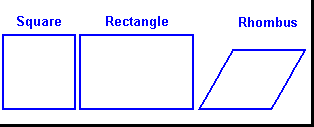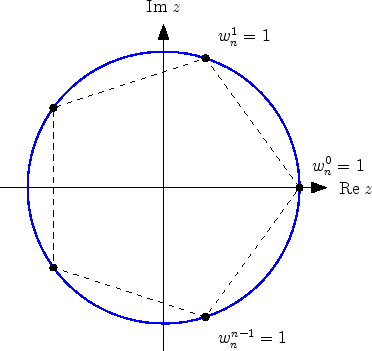## Friday, April 23, 2010

### Polytopes: Part I

Polytopes, simply defined, are geometrical shapes. They can exist in any dimension, and are usually finite. For the purpose of the post, all polytopes are considered finite unless specifically designated to the contrary.

Starting in zero dimensions, there is no direction, and the only thing that can exist is an infinitesimal speck, called a point. In one dimension, an infinite number of distinct points exist. By connecting any two of these points, a line segment is formed.A point and a line representing zero and one dimensions.

In abstract mathematical space the line must continue forever, representing the entire one-dimensional universe.

In two dimensions, there are an infinite number of lines possible, all crisscrossing what is called a plane. We are familiar with many shapes, called polygons, on this plane. One and two sided polygons aren't really possible with straight line segments, but one and two sided polygons with arcs of a circle as sides, called henagons and digons are technically possible. The simplest real polygon however, is the triangle. A triangle is the shape defined by the intersections of three distinct nonparallel line segments. A picture of a triangle is shown below.A triangle. The line segments are called the sides, and each pair of sides marks an angle. The triangle is the interior region defined by three line segments, and including the line segments. The rest of the plane is known as the exterior.

For a triangle, if it is equilateral, or all the sides are the same length, it follows that the triangle is also equiangular, or that the angles all have the same measure. This only follows for the triangle and not any higher polygon. The triangle that is both equiangular and equilateral is called a regular triangle. It is given the symbol {3}. The angles of any triangle in regular space must add up to 180º, with a general Euclidean space polygon's angles add up to 180º(p-2) where p is the number of sides in the polygon.

Moving on, one can define a polygon with four sides, known as a quadrilateral. From the formula mentioned above, it is clear that the angles of a quadrilateral add up to 360º. With four sides, a polygon can be equilateral without be equiangular, the example being the rhombus, and can also be equiangular without being equilateral, the example being the rectangle. The two shapes are shown below, along with the quadrilateral that is both equilateral and equiangular, the square, which is denoted by {4}.All of the above polygons mentioned are convex, meaning that none of their angles' measures exceed 180º. However, with polygons of four sides and up, concave polygons exist, and these can even intersect themselves. When a polygon intersects itself on the plane, it is known as a complex polygon.The above is a concave quadrilateral, followed by a complex one.

In two dimensions, a polygon with any number of sides can exist and the regular convex pentagon {5}, regular convex hexagon {6}, regular convex heptagon {7}... etc. follow the quadrilateral. In general a regular convex polygon {p} has p points, or vertices, and p line segments, or sides. The total angular measure of a regular convex polygon is 180º(p-2), and each individual angle is 180(1-2/p), however, the sum of exterior angles of any regular convex polygon is always 360º.

A polygon with p sides can also be generated by taking the pth roots of one, and plotting them on the complex plane. Each vertices' coordinates is found with (cos(2πik/p),sin(2πik/p), where k is {0,1,2,3...p-1}. The pentagon found be connecting the 5th roots of one is shown belowNote that all five points lie on the unit circle, or the circle with radius one centered at the origin (the point (0,0)).

However, a regular polygon does not have to be convex. There are regular polygons which are complex. These are known as star polygons. Each star polygon with p sides has the same vertices as a regular convex polygon {p}, but the sides are connected in a different way. The simplest star polygon is known as the pentagram, and is shown below.This particular star polygon is denoted {5/2} because the points are connected in such a way that every point is connected to the point two points away from it in a counterclockwise order. With five sides, {5/1} simply connects the points in order, simply resulting in {5}, {5/3} is a simple reflection of {5/2} and {5/4} is just the points connected in the opposite order, still resulting in {5}.

In the case of {6}, no continuous star polygon can exist, because {6/1} and {6/5} trivially equal {6}, and the remaining three, {6/2}, {6/3} and {6/4} are not continuous, meaning that despite what point of the hexagon one starts at, not all the points are accessed, and the polygon is made up of multiple parts, two for {6/2} (the Jewish star) and {6/4}, for which the parts are triangles, and three for {6/3}, the parts being lines.The star polygon {6/2}. It is made up of two disconnected triangles, and is therefore not a true star polygon.

The number of distinct continuous star polygons, disregarding simple reflections and rotations, for all p up to 10 are

p=1-4: 0
p=5: 1; {5/2}
p=6: 0
p=7: 2 {7/2}, {7/3}
p=8: 1; {8/3}
p=9 2; {9/2}, {9/4}
p=10 1; {10/3}

All possible star polygons with p up to 16 are shown below (note p is replaced by n in the picture).In the above picture, the red diagonal lines represent {p/1} which is simply {p}, {p/2}, {p/3} and so on and the blue diagonal lines show the common names for these star polygons. Note that the continuous star polygons are in boldface, while the discontinuous ones are not (click on the image to enlarge it).

In conclusion, a star polygon {p/x} is continuous when GCD(p,x)=1 (greatest common divisor of x and p). An equivalent statement is that p and x are relatively prime. GCD(p,x) is also the number of discontinuous elements required to include all the points. For example, since GCD(5,2)=1, {5/2} is a continuous star polygon, but GCD(6,2)=2 and two distinct pieces (in this case triangles) are required to include all the points with a {6/2} polygon. Additionally, if p-x=±1, then {p/x} is equivalent to {p} and is a special case of the more general star polygon, the convex polygon.

A final notable case of polygon is the star-shaped polygon, which is very different from a star polygon. For any star-shaped polygon, there exists one or more points on the interior of the polygon from which a line can be drawn to each vertex without intersecting any other part of the polygon. An example of a star polygon is shown below.The top image shows how the polygon's interior contains at least one point from which all vertices are visible, or accessible with lines without obstruction. In addition, the set of points where this is true is named with the (quite odd) name, the kernel. The lower image shows the kernel of this particular star-shaped polygon. Complex polygons cannot be star-shaped, and, ironically, nor can star polygons. Convex polygons are all star-shaped, and their kernels coincide with the polygons themselves. Additionally, all triangles are star-shaped by definition, because they all are convex.

This post is continued in Polytopes Part II.

Sources: Regular Polytopes by H.S.M. Coxeter, Google Images

#### 1 comment:

Noah Elkins said...

Whoa! I sincerely loved your diagnosis on pentagrams. They are the most interesting of all five-pointed geometric figures.